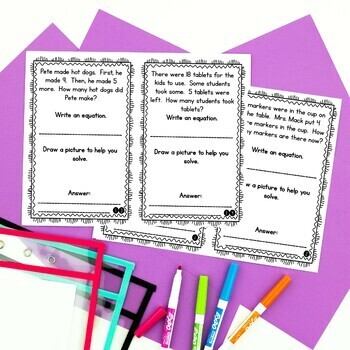# 1st Grade Word Problem of the Day Story Problems- BundleSubject
Resource Type
Format
Zip (20 MB|288 pages)
Standards
\$21.00
Bundle
List Price:
\$27.00
You Save:
\$6.00
\$21.00
Bundle
List Price:
\$27.00
You Save:
\$6.00
Products in this Bundle (10)

showing 1-5 of 10 products

• Bundle Description
• Standards

These 1st Grade Word Problem of the Day word problems contain real-world applications of the first grade math standards. Students build problem solving skills through guided and independent practice of the standards daily. This nine month 1st Grade Word Problem bundle includes 32 story problems for each month of the school year (Sept-May) that build students' problem solving skills through guided and independent practice of the standards daily.

Skills included:

• addition and subtraction within 10

• addition and subtraction within 20 with unknowns in all positions

• using money (dollar amounts and with only pennies)

• adding three numbers within 20

• comparative story problems

• writing number sentences

• completing part-part-whole tables

• completing start-change-end tables

• creating tables

• drawing a picture to represent the problem

• writing answers in complete sentences

• addition and subtraction within 100 without regrouping

Each month includes two files: each problem printed twice per page in half sheets, or problems printed two per page in half sheets. 32 pages are provided each month so that a page can be completed each day, if you wish, with additional pages going home for independent practice. These can be easily printed and stapled for daily use, or these are also perfect for inclusion in interactive notebooks.

The 1st Grade Word Problems of the Day:

Sept | Oct | Nov | Dec | Jan | Feb | March | April | May

You can also check out the Back to School Freebie.

CLICK HERE to follow me and be notified of future products as soon as they are posted.

_______________________________________________________________

You may not redistribute, edit, sell, or otherwise post this product on the internet. You may, however, post a link for others topurchase themselves.

to see state-specific standards (only available in the US).
Determine the unknown whole number in an addition or subtraction equation relating three whole numbers. For example, determine the unknown number that makes the equation true in each of the equations 8 + ? = 11, 5 = ▯ - 3, 6 + 6 = ▯.
Add and subtract within 20, demonstrating fluency for addition and subtraction within 10. Use strategies such as counting on; making ten (e.g., 8 + 6 = 8 + 2 + 4 = 10 + 4 = 14); decomposing a number leading to a ten (e.g., 13 - 4 = 13 - 3 - 1 = 10 - 1 = 9); using the relationship between addition and subtraction (e.g., knowing that 8 + 4 = 12, one knows 12 - 8 = 4); and creating equivalent but easier or known sums (e.g., adding 6 + 7 by creating the known equivalent 6 + 6 + 1 = 12 + 1 = 13).
Relate counting to addition and subtraction (e.g., by counting on 2 to add 2).
Understand subtraction as an unknown-addend problem. For example, subtract 10 – 8 by finding the number that makes 10 when added to 8.
Apply properties of operations as strategies to add and subtract. If 8 + 3 = 11 is known, then 3 + 8 = 11 is also known. (Commutative property of addition.) To add 2 + 6 + 4, the second two numbers can be added to make a ten, so 2 + 6 + 4 = 2 + 10 = 12. (Associative property of addition.)
Total Pages
288 pages
N/A
Teaching Duration
1 Year
Report this Resource to TpT
Reported resources will be reviewed by our team. Report this resource to let us know if this resource violates TpT’s content guidelines.
• Ratings & Reviews
• Q & A

Teachers Pay Teachers is an online marketplace where teachers buy and sell original educational materials.